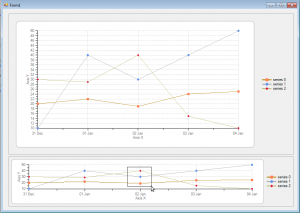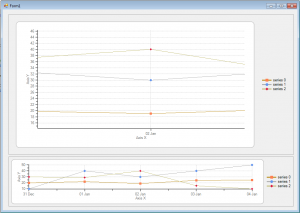﻿ Zooming using Multiple Charts in C1Chart2D# Zooming using Multiple Charts in C1Chart2D

C1Chart for Winforms has been a popular control for displaying dynamic 2D charts for any type of charting application. Objective of this blog is to provide easy to implement, Zooming effect using two C1Chart controls wherein the data region selected in the first chart will be displayed as zoomed out version in the second chart. To begin with, you need to load same data in both the charts through the LoadChartFromFile method of C1Chart object as follows :

``````
private void Form1_Load(object sender, EventArgs e)
{
c1Chart1.SaveChartToFile("Chart1_Data.xml");
}

``````

Now you need to handle the following Mouse events:

• MouseDown
• MouseMove
• MouseUp

Using these events, we will capture the Data points for the region selected by the user in the first chart. You can use the CoordToDataCoord method to convert the mouse coordinates to Data points.

``````
private void c1Chart1_MouseUp(object sender, MouseEventArgs e)
{
double X = -1, Y = -1;
if (c1Chart1.ChartGroups.CoordToDataCoord(e.X, e.Y, ref X, ref Y))
{
// data coordinates of the rectangular area selected
// at mouse up - mux,muy
mux = X;
muy = Y;
}

// Set the axis x & axis y's min & max points
if (rect.Width > 0 && rect.Height > 0)
{
c1Chart2.ChartArea.AxisY.Max = y;
c1Chart2.ChartArea.AxisX.Min = x;
c1Chart2.ChartArea.AxisX.Max = mux;
c1Chart2.ChartArea.AxisY.Min = muy;
}
rect.Size = new Size(0, 0);
}

``````
``````
private void c1Chart1_MouseDown(object sender, MouseEventArgs e)
{
rect.Size = new Size(0, 0);
double X = -1, Y = -1;
if (c1Chart1.ChartGroups.CoordToDataCoord(e.X, e.Y, ref X, ref Y))
x = X;
y = Y;

// See if the mouse down data coordinates lie in the plot area or not
if ((x >= c1Chart1.ChartArea.AxisX.Min && x <= c1Chart1.ChartArea.AxisX.Max) && (y >= c1Chart1.ChartArea.AxisY.Min && y <= c1Chart1.ChartArea.AxisY.Max))
{
flag = 1;
// data coordinates of the rectangular area selected
// at mouse down - mdx,mdy
mdx = e.X;
mdy = e.Y;
}
else
{
flag = 0;
}
}

``````
``````
private void c1Chart1_MouseMove(object sender, MouseEventArgs e)
{
if (e.Button == MouseButtons.Left)
{
if (flag == 1)
{
// Draws the rectangle as the mouse moves
rect = new Rectangle(mdx, mdy, e.X - rect.Left, e.Y - rect.Top);
}
else
{
// If the mouse down data coordinates do not lie in the plotting area then don not draw           the rectangle
rect.Size = new Size(0, 0);
}
}
c1Chart1.Invalidate();
}

``````
``````
// Draw the rectangle on the first chart as the mouse is being moved to select a specific area
private void c1Chart1_Paint(object sender, PaintEventArgs e)
{
if (flag == 1)
{
c1Chart1.Invalidate();
using (Pen pen = new Pen(Color.Black, 1))
{
e.Graphics.DrawRectangle(pen, rect);
}
}
}

``````

The final output would look as shown in the bottom image:Refer to the attached samples for the detailed implementation. Download C# Sample Download VB SampleGrapeCity

GrapeCity Developer Tools